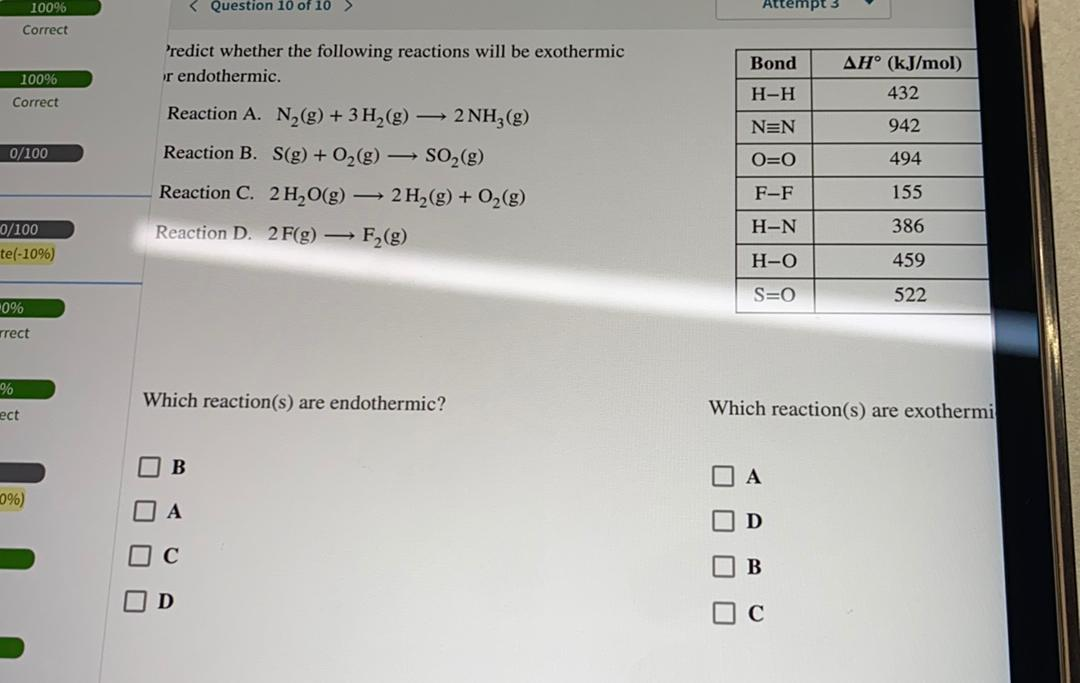Question:

Question 10 of 10 > Attempt 3 100% Correct Predict whether the following reactions will be exothermic or endothermic. Bond 10096Question 10 of 10 > Attempt 3 100% Correct Predict whether the following reactions will be exothermic or endothermic. Bond 10096 Correct AH° (kJ/mol) 432 H-H | | NEN 942 0/100 O=0 Reaction A. N2(g) + 3H2(g) — 2NH3(g) Reaction B. S(g) + O2(g) → SO2(g) Reaction C. 2 H2O(g) — 2H2(g) + O2(g) Reaction D. 2 F(g) — F (9) 494 F-F 155 H-N 386 0/100 te(-10%) H-O 459 S=O 522 0% rrect % Which reaction(s) are endothermic? Which reaction(s) are exothermi ect B 096) A D O O O O O C B D